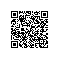# Python学习(8)--for循环1.for循环2.range函数

[python] view plain copy

1. a=range(10)
2. print(a)
3. print(a)
4. print(a)
5. print(a)
6. print(a)
7. print(a)
8. print(a)
9. print(a)
10. print(a)
11. print(a)[python] view plain copy

1. for x in range(10):
2.     print(x,"hello world")3.range函数的拓展功能

range()函数实际上可以使用三个参数来创建序列,即range(i,j,N),在python3中如果这三个参数都写,则会创建一个长度为j-i,步长为N的序列,i和j分别指定了序列的初始值和终止值,这个序列的下标是从0~j-i-1,元素为i~j-1,一共j-i个元素,N为步长,在这里我们又提到了步长这个概念,这个步长的含义其实和之前的步长是一致的,即每间隔N-1个序列元素取一个元素,来作为新序列的元素.代码如下:

[python] view plain copy

1. a=range(2,10,2)
2. for x in range(2,10,2):
3.     print(x)4.通过下标迭代列表

[python] view plain copy

1. s=["H",'e','l','l','o']
2. for x in s:
3.    print(x)

[python] view plain copy

1. s=["H",'e','l','l','o']
2. for x in range(len(s)):
3.     if x%2==0:
4.         print(s[x])5.for循环迭代字典

[python] view plain copy

1. d1={'name':'lisi','age':12,'sex':'man'}
2. print(d1.items())[python] view plain copy

1. k,v= ('age'12)
2. print(k)
3. print(v)[python] view plain copy

1. d1={'name':'lisi','age':12,'sex':'man'}
2. for k,v in d1.items():
3.     print(k,v)使用钉钉扫一扫加入圈子
+ 订阅